International
Tables for
Crystallography
Volume A
Space-group symmetry
Edited by M. I. Aroyo

International Tables for Crystallography (2016). Vol. A, ch. 1.3, pp. 22-23

## Section 1.3.2.1. Basic properties of lattices

B. Souvigniera*

aRadboud University Nijmegen, Faculty of Science, Mathematics and Computing Science, Institute for Mathematics, Astrophysics and Particle Physics, Postbus 9010, 6500 GL Nijmegen, The Netherlands
Correspondence e-mail: souvi@math.ru.nl

#### 1.3.2.1. Basic properties of lattices

| top | pdf |

The two-dimensional vector space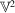is the space of columns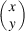with two real components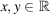and the three-dimensional vector space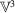is the space of columns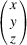with three real components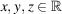. Analogously, the-dimensional vector spaceis the space of columns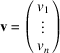with n real components.

For the sake of clarity we will restrict our discussions to three-dimensional (and occasionally two-dimensional) space. The generalization to n-dimensional space is straightforward and only requires dealing with columns of n instead of three components and with bases consisting of n instead of three basis vectors.

#### Definition

For vectorsforming a basis of the three-dimensional vector space, the set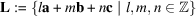of all integral linear combinations of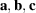is called a lattice inand the vectorsare called a lattice basis of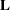.

It is inherent in the definition of a crystal pattern that the translation vectors of the translations leaving the pattern invariant are closed under taking integral linear combinations. Since the crystal pattern is assumed to be discrete, it follows that all translation vectors can be written as integral linear combinations of a finite generating set. The fundamental theorem on finitely generated abelian groups (see e.g. Chapter 21 in Armstrong, 1997) asserts that in this situation a set of three translation vectorscan be found such that all translation vectors are integral linear combinations of these three vectors. This shows that the translation vectors of a crystal pattern form a lattice with lattice basisin the sense of the definition above.

By definition, a lattice is determined by a lattice basis. Note, however, that every two- or three-dimensional lattice has infinitely many bases.

#### Example

The square lattice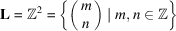inhas the vectors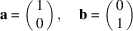as its standard lattice basis. But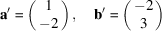is also a lattice basis of: on the one hand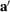andare integral linear combinations of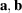and are thus contained in. On the other hand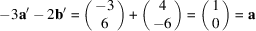and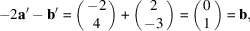henceandare also integral linear combinations of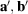and thus the two basesandboth span the same lattice (see Fig. 1.3.2.1).Figure 1.3.2.1 | top | pdf |Conventional basisand a non-conventional basisfor the square lattice.

The example indicates how the different lattice bases of a latticecan be described. Recall that for a vector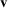=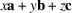the coefficientsare called the coordinates and the vectoris called the coordinate column ofwith respect to the basis. The coordinate columns of the vectors inwith respect to a lattice basis are therefore simply columns with three integral components. In particular, if we take a second lattice basisof, then the coordinate columns of,,with respect to the first basis are columns of integers and thus the basis transformationsuch that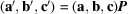is an integral 3 × 3 matrix. But if we interchange the roles of the two bases, they are related by the inverse transformation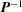, i.e.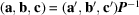, and the argument given above asserts thatis also an integral matrix. Now, on the one hand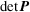and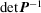are both integers (being determinants of integral matrices), on the other hand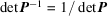. This is only possible if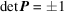.

Summarizing, the different lattice bases of a latticeare obtained by transforming a single lattice basiswith integral transformation matricessuch that.

### References

Armstrong, M. A. (1997). Groups and Symmetry. New York: Springer.Google Scholar